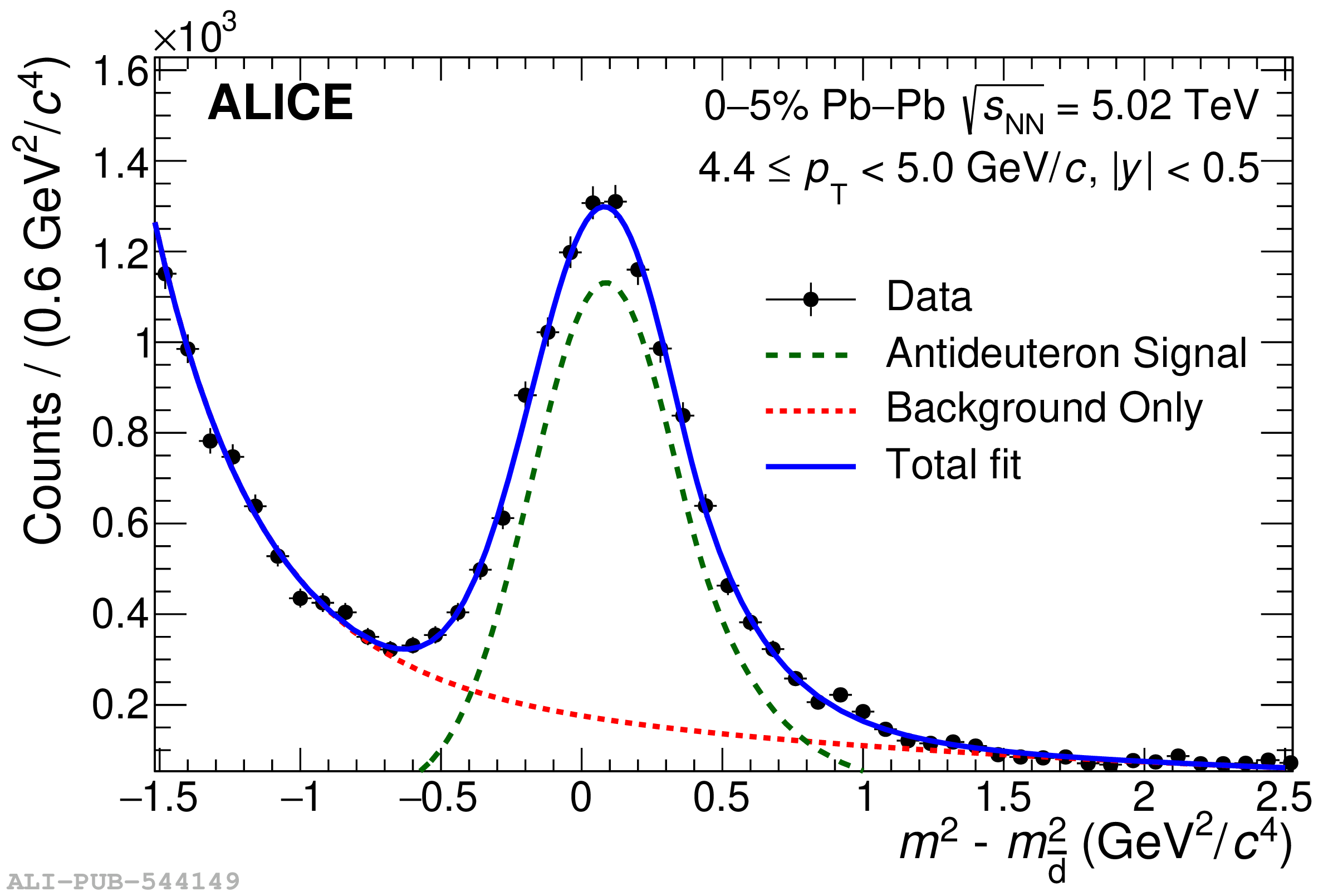# Figure 2

 Fit to the measured squared mass to extract the antideuteron signal in 4.4 $<$ \pt $<$ 5.0 GeV/$c$ (left) and the antitriton signal in 2.0 $<$ \pt $<$ 2.4 GeV/$c$ (right). The red dashed line shows the background, the solid blue line the combined fit to the data and the green dashed line the signal only.